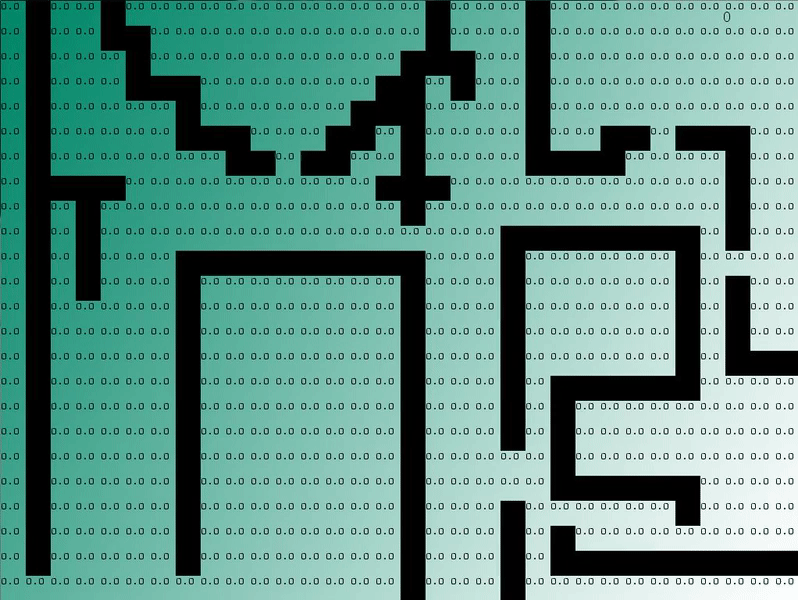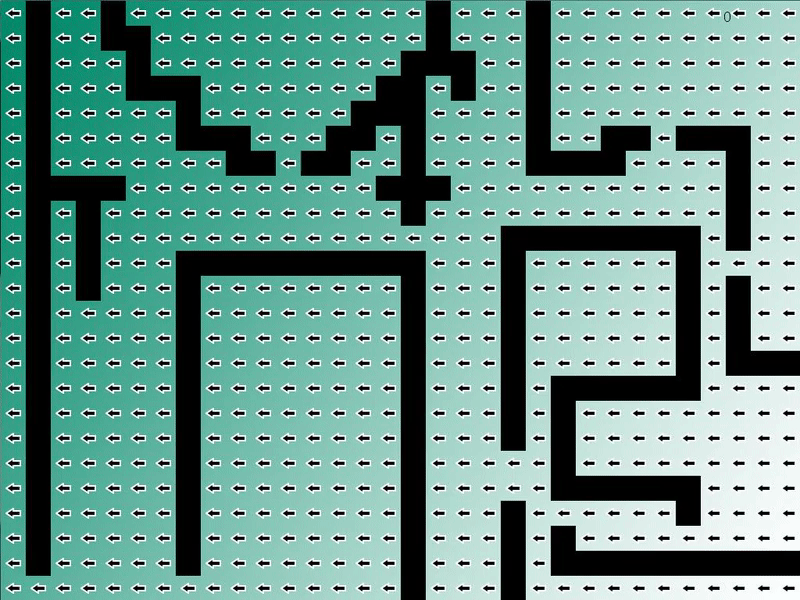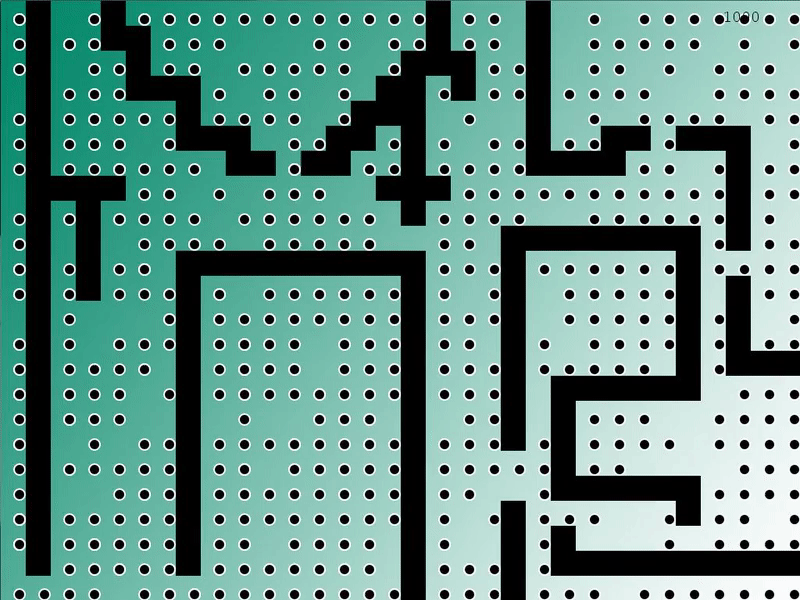# Flow Field Pathfinding

### What is Flow Field Pathfinding?

To start, I am going to assume you are familiar with the concept of Pathfinding and the more basic algorithms like Dijkstra’s and A*.

Flow field pathfinding can be classified as goal based pathfinding, which is different from Dijkstra and A*, which are A to B pathfinding. In goal based pathfinding, you are finding a path from one point to every other point on the map, without actually marking down a specific path (from point A to point B).

Flow field pathfinding is commonly used in Real Time Strategy games like Supreme Commander 2 because of its ability to handle large amounts of units all at once. The difference stems from the amount of pathfinders required in each pathfinding algorithm.

For A to B pathfinding algorithms like A*, one pathfinder for each unit is required, making it tedious and difficult if the unit count eclipses a few dozen or a few hundreds, depending on your hardware.

However, with a flow field pathfinding algorithm, only one pathfinder is required, allowing for thousands of units to exist on the screen with little calculation time in between deciding where they will be directed to.

### How is it done?

Flow field pathfinding is done through three steps:

1. Generate a heat map. This is effectively a distance calculation, wherein each square on the grid is given a “cost” of how far away it is from the goal square.
2. Generate the resulting vector field. This is done by looking at all adjacent squares and creating a vector in the direction of the one with the lowest cost.
3. Move the units along the vector field. This is as simple as having the units move in the direction of the vector designated to the square they are currently on.

### Generating the Heat Map

My implementation of the heat map is very similar to Dijkstra’s, altering out the part where it returns a specific path.

The algorithm follows these key ideas:

1. Sets all nodes to unvisited
2. Sets the goal node cost to 0 and open
3. Cycles through all open nodes, finding the lowest cost node
4. Adds any adjacent nodes that have not been visited to the open list
5. After all adjacent nodes are checked and opened if necessary, it closes the current node and moves back to step 3
```void FlowField::generateHeatMap(Node* pFrom)
{
//Declare all squares as unvisited
for (size_t i = 0; i < GRID_TOTAL; i++)     {           mNodeArray[i].status = UNVISITED;      }      //Set the cost of the goal to 0 and set as open      mNodeArray[pFrom->getId()].cost = 0;
mNodeArray[pFrom->getId()].status = OPEN;

int openCount = getOpenCount();
int arrayPosition;

while (openCount > 0)
{
float min = 9999;
//Find the lowest cost square that is open
for (size_t i = 0; i < GRID_TOTAL; i++)
{
if (mNodeArray[i].cost < min && mNodeArray[i].status == OPEN)
{
min = mNodeArray[i].cost;
arrayPosition = i;
}
}

vector<Connection*> connections =
mpGraph->getConnections(mNodeArray[arrayPosition].node->getId());

for (unsigned int i = 0; i < connections.size(); i++)          {              Connection* pConnection = connections[i];               Node* endNode = pConnection->getToNode();
float endCost = mNodeArray[arrayPosition].cost +
pConnection->getCost();

bool found = false;

//Check if the connected square has been visited
for (size_t i = 0; i < GRID_TOTAL; i++)               {                   if (mNodeArray[i].status != UNVISITED &&                       mNodeArray[i].node->getId() == endNode->getId())
{
found = true;
break;
}
}
//If not, declare it open and set its cost
if (!found)
{
mNodeArray[endNode->getId()].connection = pConnection;
mNodeArray[endNode->getId()].cost = endCost;
mNodeArray[endNode->getId()].status = OPEN;
}
}
//After cycling through all the connections, close the current square
mNodeArray[arrayPosition].status = CLOSED;
openCount = getOpenCount();
}
}```### Generating the Vector Field

The vector field is less code, but accomplishes the same steps:

1. Cycling through the grid
2. Checking all the adjacent squares
3. Finding the one with the lowest cost
4. Setting the direction vector toward the adjacent square with the lowest cost
```void FlowField::generateVectorField(Node* pFrom)
{
//Cycle through all squares
for (int i = 0; i < GRID_TOTAL; i++)
{
vector<Connection*> connections =
mpGraph->getConnections(mNodeArray[i].node->getId());

int min = 9999, nodeNum = 0;
for (unsigned int i = 0; i < connections.size(); i++)          {              Connection* pConnection = connections[i];              //Check all adjacent squares for the lowest value and make sure              // that it's not an untraversable square (blocking value)              if (mNodeArray[pConnection->getToNode()->getId()].cost <= min &&                  pGame->getGrid()->getValueAtIndex(pConnection->getToNode()->
getId()) != BLOCKING_VALUE)
{
min = mNodeArray[pConnection->getToNode()->getId()].cost;
nodeNum = pConnection->getToNode()->getId();
}
}
//As long as the square is traversable, set the direction
if (pGame->getGrid()->getValueAtIndex(i) != BLOCKING_VALUE)
{
mNodeArray[i].direction = Vector2D(pGame->getGrid()->
getULCornerOfSquare(i, true) -
pGame->getGrid()->getULCornerOfSquare(nodeNum, true));
}
//Otherwise, give it a zero value
else
{
mNodeArray[i].direction = ZERO_VECTOR2D;
}
}
//Save the goal node index for moving units
endIndex = pFrom->getId();
}```### Moving the Units

Moving the units is a rather straight forward situation, where you have each unit move at a velocity that is designated by the directional vector of the node they are standing on.

However, when moving my units, I ran into an issue where because the entire square acted as a directional “hot zone,” where whenever a unit entered it it would default to that direction, I had to figure out a way to move around walls, as my units would run into them frequently. My solution is far from elegant, but gets the job done.

I ended up going with an algorithm that changes the units direction when within a 20 pixel block of the center of the square, and otherwise it defaults to the last direction it was currently moving.

The unfortunate side effect of this is that it results in the units looking like they are traversing through walls when in fact they’re following the direction they were last given before running into a wall square.

```void Unit::update(GameApp* pGame)
{
Grid* grid = pGame->getGrid();

//Check if the current square is the goal node
if (grid->getSquareIndexFromPixelXY(mPos.getX(), mPos.getY()) !=
pGame->getPathfinderManager()->getPathfinders()->getEndIndex())
{
Vector2D move;
float num = 20;

//Check if the position of the unit is within num-sized block
if (mPos.getX() <= grid->getULCornerOfSquare(
grid->getSquareIndexFromPixelXY(mPos.getX(), mPos.getY()),
true).getX() + num && mPos.getX() >= grid->getULCornerOfSquare(
grid->getSquareIndexFromPixelXY(mPos.getX(), mPos.getY()),
true).getX() - num && mPos.getY() <= grid->getULCornerOfSquare(
grid->getSquareIndexFromPixelXY(mPos.getX(), mPos.getY()),
true).getY() + num && mPos.getY() >= grid->getULCornerOfSquare(
grid->getSquareIndexFromPixelXY(mPos.getX(), mPos.getY()),
true).getY() - num)
{
//If its a wall, continue moving in the last direction given
if (grid->getValueAtPixelXY(mPos.getX(), mPos.getY()) ==
BLOCKING_VALUE)
{
move = lastDir;
}
//Otherwise find the new direction of the node underneath the unit
else
{
move = Vector2D(-pGame->getPathfinderManager()->
getPathfinders()->getNodeArray()
[grid->getSquareIndexFromPixelXY(mPos.getX(), mPos.getY())]
.direction.getX(),
-pGame->getPathfinderManager()->getPathfinders()->
getNodeArray()[grid->getSquareIndexFromPixelXY
(mPos.getX(), mPos.getY())].direction.getY());
lastDir = move;
}
}
//Normalize, set the direction, and move the unit
move.normalize();
move *= VELOCITY;
mPos += move;
}
}```### Tech Demo

If you are interested in trying out the program for yourself, it can be found here.

### References

Emerson, Elijah. “Crowd Pathfinding and Steering Using Flow Field Tiles.” Game AI Pro: Collected Wisdom of Game AI Professionals. Boca Raton: CRC, 2014. 307-23. Print.

Erkenbrach, Leif. “Flow Field Pathfinding.” Leif Node. WordPress, 5 Dec. 2013. Web. 11 Dec. 2016.

Leaver, Dave. “Basic Flow Fields.” Blog post. How to RTS: Basic Flow Fields. N.p., 4 Jan. 2014. Web. 11 Dec. 2016.